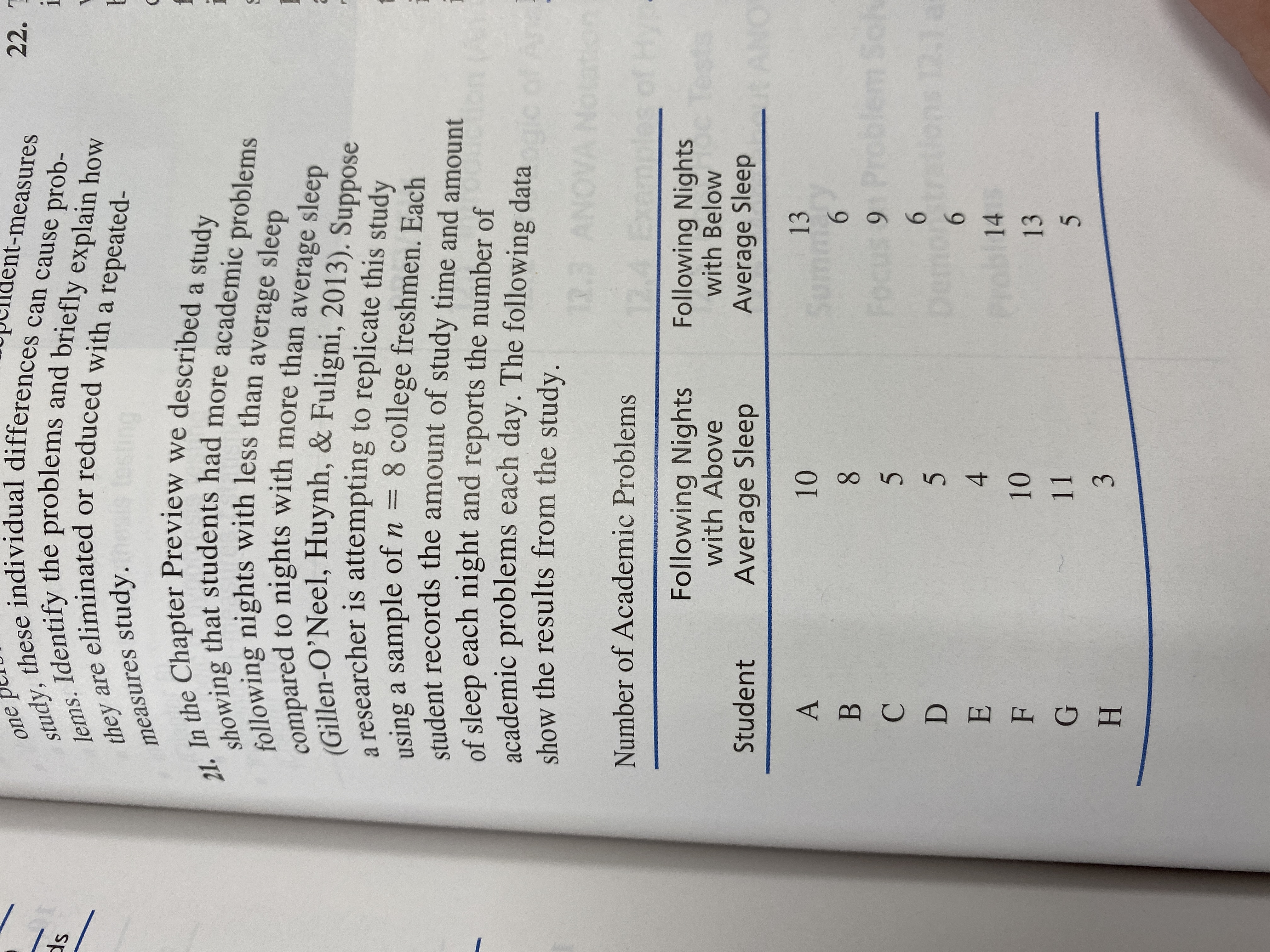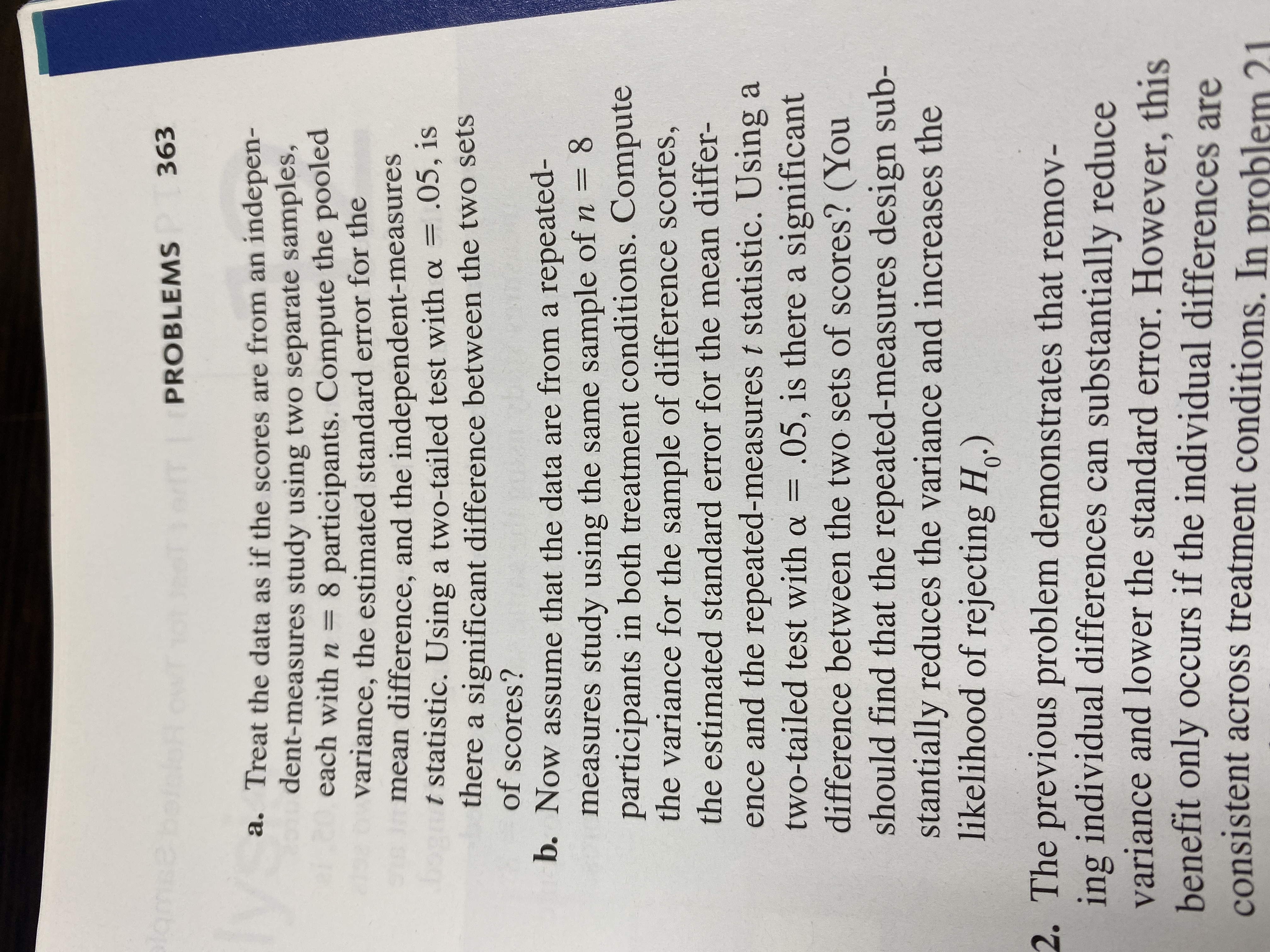# ent-measuresstudy, these individual differences can cause prob-lems. Identify the problems and briefly explain howthey are eliminated or reduced with a repeated-22. TOnedsmeasures study.21. In the Chapter Preview we described a studyshowing that students had more academic problemsfollowing nights with less than average sleepcompared to nights with more than average sleep(Gillen-O'Neel, Huynh, & Fuligni, 2013). Supposeo researcher is attempting to replicate this studyusing a sample of n 8 college freshmen. Eachstudent records the amount of study time and amountof sleep each night and reports the number ofacademic problems each day. The following datashow the results from the study.R.3 ANOVANdtorNumber of Academic Problems12Exees of HyFollowing Nightswith AboveFollowing Nightswith Belowe TestsAverage SleepStudentAverage Sleep1310АSuCy68ВSolt9 ProblerFocus612.1emp6alD4EProb1410F131153H lar363PROBLEMSa. Treat the data as if the scores are from an indepen-dent-measures study using two separate samples,each with n8 participants. Compute the pooledvariance, the estimated standard error for themean difference, and the independent-measurest statistic. Using a two-tailed test with o=there a significant difference between the two setsof scores?.05, isb. Now assume that the data are from a repeated-8measures study using the same sample of n =participants in both treatment conditions. Computethe variance for the sample of difference scores,the estimated standard error for the mean differ-ence and the repeated-measures t statistic. Using atwo-tailed test with a .05, is there a significantdifference between the two sets of scores? (Youshould find that the repeated-measures design sub-stantially reduces the variance and increases thelikelihood of rejecting Ho.)2. The previous problem demonstrates that remov-ing individual differences can substantially reducevariance and lower the standard error. However, thisbenefit only occurs if the individual differences areconsistent across treatment conditions. In problem 2.1

Question
14 views

Number 21help_outlineImage Transcriptioncloseent-measures study, these individual differences can cause prob- lems. Identify the problems and briefly explain how they are eliminated or reduced with a repeated- 22. T One ds measures study. 21. In the Chapter Preview we described a study showing that students had more academic problems following nights with less than average sleep compared to nights with more than average sleep (Gillen-O'Neel, Huynh, & Fuligni, 2013). Suppose o researcher is attempting to replicate this study using a sample of n 8 college freshmen. Each student records the amount of study time and amount of sleep each night and reports the number of academic problems each day. The following data show the results from the study. R.3 ANOVANd tor Number of Academic Problems 12 Exe es of Hy Following Nights with Above Following Nights with Below e Tests Average Sleep Student Average Sleep 13 10 А Su Cy 6 8 В Solt 9 Probler Focus 6 12.1 emp 6 al D 4 E Prob 14 10 F 13 11 5 3 H fullscreenhelp_outlineImage Transcriptioncloselar 363 PROBLEMS a. Treat the data as if the scores are from an indepen- dent-measures study using two separate samples, each with n 8 participants. Compute the pooled variance, the estimated standard error for the mean difference, and the independent-measures t statistic. Using a two-tailed test with o= there a significant difference between the two sets of scores? .05, is b. Now assume that the data are from a repeated- 8 measures study using the same sample of n = participants in both treatment conditions. Compute the variance for the sample of difference scores, the estimated standard error for the mean differ- ence and the repeated-measures t statistic. Using a two-tailed test with a .05, is there a significant difference between the two sets of scores? (You should find that the repeated-measures design sub- stantially reduces the variance and increases the likelihood of rejecting Ho.) 2. The previous problem demonstrates that remov- ing individual differences can substantially reduce variance and lower the standard error. However, this benefit only occurs if the individual differences are consistent across treatment conditions. In problem 2.1 fullscreen
check_circle

Step 1

Independent-measures t test:

Denote μ1, μ2 as the true average number of academic problems on nights after below average sleep, and under above average sleep, respectively. The null and alternative hypotheses are as follows:

H0: μ1 = μ2 versus H1: μ1 ≠ μ2.

The two-sample t test is to be conducted, at level of significance α = 0.05.

We have entered the data in an Excel sheet, with the data on below average sleep in column A, and that on above average sleep in column B. The first row has been used for labels.

The respective sample means are, 1 = 9 and 2 = 7 [Using Excel formulae: =AVERAGE(A2:A9) and =AVERAGE(B2:B9) respectively].

The sample variances are respectively, s12 = 14.28571, s22 = 9.71429 [Using Excel formulae: =VAR.S(A2:A9) and =VAR.S(B2:B9) respectively].

Here, n1 = n2 = 8

The degrees of freedom is, df = n1 + n2 – 2 = 8 + 8 – 2 = 14.

The pooled variance is:

s2 = [(n1 – 1) s12 + (n2 – 1) s22] / (n1 + n2 – 2)

= [(7 × 14.28571) + (7 × 9.71429)] / 14

= 12.

Thus, pooled variance = 12.

The standard error for the mean difference is:

se = s√ (1/n1 + 1/n2)

= √[s2(1/n1 + 1/n2)]

= √ [12 (1/8 + 1/8)

= 1.7321.

Thus, standard error of mean difference = 1.7321.

The test statistic is found as follow...

### Want to see the full answer?

See Solution

#### Want to see this answer and more?

Solutions are written by subject experts who are available 24/7. Questions are typically answered within 1 hour.*

See Solution
*Response times may vary by subject and question.
Tagged in

### Hypothesis Testing